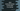# Java program to move all zeroes of an integer array to the start### Java program to move all zeros of an integer array to the start of an array :

In this tutorial, we will learn how to move all zeros of an array to the start of it using Java programming language. For example, for the array `{1,0,2,0,3,0}`, it will become `{0,0,0,1,2,3}` after the conversion.

### Algorithm :

We will modify the original array. The program will iterate over the elements of the array from right to left and fill it with non-zero elements from the end. Once the non-zero elements are filled, it will fill the remaining positions with zero.

1. The program will iterate over the elements of the array in reverse i.e. from the end index to the start index of the array. For example, for the array {1,0,2,0,3,0}, it will iterate the elements as 0,3,0,2,0 and 1.

2. Create one variable to store the current index to fill the array. Before the program starts, its value is equal to the last index of the array i.e. the first non-zero element is added at the end of the array.

3. We need to check each element of the array while iterating over them. If the element is not zero, assign the value at the array index position defined by the current index variable.

4. After the iteration is completed, fill the remaining position of the array with 0.

5. Finally, print out the array.

### Method 1: Java Program to move the zeros to the start of an array by keeping the order:

The following program shows how to move the zeroes of an integer array to the start of the array:

``````class Main {
public static void main(String args[]) {
// 1
int[] array = { 1, 2, 0, 4, 6, 0, 9, 0, 4, 0, 3, 0, 9, 0, 1, 0, 3, 0 };

// 2
int current = array.length - 1;

// 3
for (int i = array.length - 1; i >= 0; i--) {
if (array[i] != 0) {
array[current] = array[i];
current--;
}
}

// 4
while (current >= 0) {
array[current] = 0;
current--;
}

// 5
for (int i = 0; i < array.length; i++) {
System.out.print(array[i] + " ");
}
}
}``````

### Explanation :

The commented numbers in the above program denote the step numbers below :

1. The array is assigned to the variable `array`.

2. It created one integer variable `current` to store the current index position of the array to assign the non-zero value. The value of the `current` variable is `array.length - 1` i.e. the last index of the array.

3. It uses one `for` loop to scan the numbers from the end of the array to the start of the array. It runs from `i = array.length - 1` to `i = 0`. For each element, we need to check if it is equal to 0 or not. If it is not equal to zero, assign the value to the array at the index position `current` and decrement the value of the `current` variable by 1. Else, continue the loop.

4. After all the elements are scanned, fill the remaining positions of the array with 0. We are using a `while` loop to fill the remaining indices. It will assign 0 to all the remaining indices.

5. Finally, print out the elements of the array.

#### Output :

Following is the output of the above program :

``0 0 0 0 0 0 0 0 1 2 4 6 9 4 3 9 1 3``

### Method 2: Java Program to move the zeros to the start of an array without keeping the order:

The above method moves the zeros to the start of an array without changing the order of the array. If we don’t have to keep the order of the elements, we can simply sort the array elements to move the zeros to the start of the array.

``````import java.util.Arrays;

public class Example {
public static void main(String args[]) {
int[] array = { 1, 2, 0, 4, 6, 0, 9, 0, 4, 0, 3, 0, 9, 0, 1, 0, 3, 0 };

Arrays.sort(array);

for (int j : array) {
System.out.print(j + " ");
}
}
}``````

We are using the `Arrays.sort` method to sort the array. We have also replaced the `for` loop with an enhanced `for` loop to iterate over the array elements. If you run this program, it will print:
``0 0 0 0 0 0 0 0 1 1 2 3 3 4 4 6 9 9 ``